# Pipes

Water pipe has a cross-section 1087 cm2. An hour has passed 960 m3 of water. How much water flows through the pipe with cross-section 300 cm2 per 9 hours if water flow same speed?

Result

V2 =  2384.5 m3

#### Solution:Leave us a comment of example and its solution (i.e. if it is still somewhat unclear...):Be the first to comment!#### To solve this example are needed these knowledge from mathematics:

Tip: Our volume units converter will help you with converion of volume units.

## Next similar examples:

1. Hectoliters of waterThe pool has a total of 126 hectoliters of water. The first pump draws 2.1 liters of water per second. A second pump pumps 3.5 liters of water per second. How long will it take both pumps to drain four-fifths of the water at the same time?
2. Barrel with water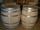The water barrel has a weight of 52 kg. When we pour a quarter of the water, the weight of the barrel is reduced to 40 kg. Determine the weight of the empty barrel.
3. Cylinder melted into cuboid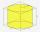A circular cylinder has area of cross section 56cm2 and the height is 10cm the cylinder is melted and made into a cuboid of base area 16cm2. What is the height of the cuboid?
4. Velocity ratio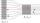Determine the ratio at which the fluid velocity in different parts of the pipeline (one part has a diameter of 5 cm and the other has a diameter of 3 cm), when you know that at every point of the liquid is the product of the area of tube [S] and the fluid.
5. Tmp workers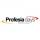Three temporary workers cleaned 60 m of walkway in 5 hours. How many meters of the walkway cleared seven volunteers for eight hours?
6. Theorem proveWe want to prove the sentence: If the natural number n is divisible by six, then n is divisible by three. From what assumption we started?
7. Tractors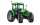Wheel and belt tractors each day plow 8 ha of fields. For plow 76 hectares, the wheel would have to work for 12 days and 8 days with the belt tractor together. How many hectares do each day of each tractor?
8. Numbers at ratio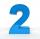The two numbers are in a ratio 3:2. If we each increase by 5 would be at a ratio of 4:3. What is the sum of original numbers?
9. Speedometer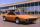The speedometer of a car reads 66 km/h. It's accurate to within 4.1% What is the maximum possible error?
10. Gas consumption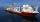The vessel consume 100 tons of gas in 250 miles. How many fuel will the vessel consume if it travels 400 miles?
11. BottlesThe must is sold in 5-liter and 2-liter bottles. Mr Kucera bought a total of 216 liters in 60 bottles. How many liters did Mr. Kucera buy in five-liter bottles?
12. Cows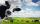4 cows spent 16 bags of hay in 5 days. How many bags of hay sacks is needed for 5 cows for seven days?
13. AP - simpleDetermine the first nine elements of sequence if a10 = -1 and d = 4
14. BlocksThere are 9 interactive basic building blocks of an organization. How many two-blocks combinations are there?
15. Reference angleFind the reference angle of each angle:
16. Ratio v2Decrease in the ratio 12:16 number 13.2.
17. RatioIncrease in the ratio 20:4 number 18.5.